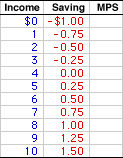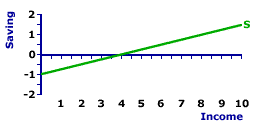Wednesday  February 1, 2023
 AmosWEB means Economics with a Touch of Whimsy!KEYNESIAN THEORY: A theory of macroeconomics developed by John Maynard Keynes built on the proposition that aggregate demand is the primary source of business cycle instability, especially recessions. The basic structure of the Keynesian theory of economics was initially presented in Keynes' book The General Theory of Employment, Interest, and Money (1936).MARGINAL PROPENSITY TO SAVE:

The proportion of each additional dollar of household income that is used for saving. The marginal propensity to save (abbreviated MPS) is another term for the slope of the saving line and is calculated as the change in saving divided by the change in income. The MPS plays a central role in Keynesian economics. It quantifies the saving-income relation, which is the flip side of the consumption-income relation, and thus it reflects the fundamental psychological law. It is also a critical to the multiplier process. A related saving measure is the average propensity to save.
The marginal propensity to save (MPS) indicates what the household sector does with extra income. The MPS indicates the portion of additional income that is used for saving. If, for example, the MPS is 0.25, then 25 percent of extra income goes for saving.

Because saving is the complement of consumption, the marginal propensity to save reflects key aspects about household consumption and saving activity. This activity is critical to the macroeconomy and the study of Keynesian economics. First, the MPS captures induced saving, which is one aspect of the fundamental psychological law of consumer spending proposed by John Maynard Keynes as a key difference between his Keynesian theory and classical economics. Second, the MPS is the slope of the saving line, which makes it the foundation for Keynesian injections-leakages model. Third, the MPS affects the multiplier process and affects the magnitude of the expenditures and tax multipliers.

### The MPS Formula

The standard formula for calculating marginal propensity to save (MPS) is:
 MPS = change in savingchange in income
This formula has a couple of interpretations.
• First, it quantifies induced saving, that is, how much of each extra dollar of income is used for saving. If income changes by \$1, then saving changes by the value of the MPS. Income induces the change in saving at a rate measured by the MPS.

• Second, the MPS is actually a measure of the slope of the saving line. The measurement of slope is generally given as the "rise" over the "run." For the saving line, the rise is the change in saving and the run is the change in income.

### A Schedule Of Numbers

Saving ScheduleA saving schedule, such as the one presented to the right, provides data that can be used to run through a few MPS calculations. The first column in this schedule presents household income, ranging from \$0 to \$10 trillion. The second column presents saving, ranging from -\$1 to \$1.5 trillion. The task at hand is to derive the marginal propensity to save at each income level.

The marginal propensity to save is calculated by dividing the change in saving in the second column by the change in income in the first column. Beginning at the top of the schedule, household income increases from \$0 to \$1 trillion. This \$1 trillion change in income induces a change in saving from -\$1 trillion to -\$0.75 trillion, a change of \$0.25 trillion.

Running the numbers through the MPS formula gives:

 MPS = change in savingchange in income = \$0.25\$1 = 0.25
Calculations for each change in income produce similar results. For example, the change in income from \$4 trillion to \$5 trillion results in a change in saving from \$0 to \$0.25 trillion. And the change in income from \$8 trillion to \$9 trillion results in a change in saving from \$1 trillion to \$1.25 trillion. In both cases, the resulting marginal propensity to save is 0.25.

In fact, a quick run through the numbers for each change in saving shows that the MPS is constant and equal to 0.25. To display all marginal propensity to save values, click the [MPS] button.

While the MPS is not necessarily constant at for all changes in income (in fact, the MPS tends to increase at higher income levels), most analysis of saving generally works with a constant MPS. It tends to make subsequent calculations for things like the multiplier a lot easier.

### The Slope Of The Line

Saving LineThe marginal propensity to save is another term for the slope of the saving line. This can be demonstrated and illustrated using the green saving line, labeled S, in the exhibit to the right. Most notable, the saving line is positively sloped, indicating that greater levels of income generate greater saving by the household sector.

This saving line reflects a plot of the numbers in the saving schedule as well as the following saving function:

 S = - 1 + 0.25Y
As specified by the saving function, the slope of this saving line is equal to 0.25. This slope value indicates that each \$1 change in income induces a \$0.25 change in saving. In general, slope is calculated as the "rise" over the "run," that is, the change in the variable on the vertical axis (saving) divided by the change in the variable on the horizontal axis (income).

The change in saving divided by the change in income is the specification of the marginal propensity to save. That is, the slope of the saving line is the marginal propensity to save. To highlight this point, click the [Slope] button in this exhibit.

Moreover, because the saving line is a straight line, the slope is constant over the entire range of income. This means that the marginal propensity to save is also constant, a conclusion reached when working through the saving schedule.

### Multiplier

While the marginal propensity to save pops up throughout the study of macroeconomics, few if any topics are more important than the multiplier. The multiplier measures the magnified change in aggregate production (gross domestic product) resulting from a change in an autonomous variable (such as investment expenditures).

The magnified change occurs because a change in production (such as what occurs when investment expenditures purchase capital goods) generates income, which then induces consumption. However, the resulting consumption is also an expenditure on production, which generates more income, which induces more consumption. This next round of consumption also triggers a change in production, which generates even more income, and which induces even more consumption.

And on it goes, round after round. The end result is a magnified, multiplied change in aggregate production initially triggered by the change investment, but amplified by the change in consumption.

The MPS enters into the process because it indicates the division of extra income between consumption and saving. It determines how much saving is induced with each change in production and income, and thus how much consumption is induced. If the MPS is smaller, then the multiplier process is also greater as less saving is induced, but more consumption is induced, with each round of activity.

This connection between the multiplier process and the marginal propensity to save is illustrated in the standard formula for a basic expenditures multiplier:

 expendituresmultiplier = 1marginal propensity to save
A decrease in the marginal propensity to save reduces the value of the denominator on the right-hand side of the equation, which then increases the overall value of the fraction and thus the size of the multiplier.

For example, a marginal propensity to save of 0.25 results in a multiplier of 4. In contrast, a smaller marginal propensity to save of 0.2 results in a larger multiplier of 5.

### Other Marginals

The marginal propensity to save is only one of several marginals that enter into the study of Keynesian economics. All induced variables have corresponding marginals that quantify the impact of income changes.

Here a few of the more important marginals:

• Marginal Propensity to Consume: The flip side of saving is consumption. The fundamental psychological law indicates that an increase in income induces changes in both consumption and saving. The marginal propensity to consume (MPC) quantifies the consumption part of this relation. It indicates the change in consumption resulting from a change in income. In fact, if the MPC and MPS are calculated based on after-tax disposable income, then the two marginals sum to one: MPC + MPS = 1.

• Marginal Propensity to Invest: Consumption is not the only one of the aggregate expenditures induced by income and with a corresponding marginal. The marginal propensity to invest (MPI) is the change in investment induced by a change in income. The induced change in investment is not nearly as big as saving, but it does affect the slope of the aggregate expenditures line and the size of the multiplier.

• Marginal Propensity for Government Purchases: Government purchases, like investment, is also induced by income and has a corresponding marginal. The marginal propensity for government purchases (MPG) is the change in government purchases induced by a change in income. The induced change in government purchases is related to the induced change in tax collections, and while it also small compared to saving, but it too affects the slope of the aggregate expenditures line and the size of the multiplier.

• Marginal Propensity to Import: The last of the four aggregate expenditures, net exports (exports minus imports), also has a corresponding marginal. However, the marginal is not for net exports proper, but for the imports part. The marginal propensity to import (MPM) is the change in imports induced by a change in income. The induced change in imports is closely connected to the marginal propensity to save. That is, a portion of saving is actually used to purchase imports, which is reflected the marginal propensity to import.

### Average Propensity to Save

The marginal propensity to save is one of two measures of the relation between saving and income. The other is average propensity to save (APS). Average propensity to save is the proportion of household income used for saving. It is found by dividing saving by income.

The formula for calculating average propensity to save (APS) looks a lot like that for the MPS, but with important differences:

 APS = savingincome
Rather than the CHANGE in saving divided by the CHANGE in income, the APS measures TOTAL saving divided by TOTAL income. In particular, the APS indicates how the household sector divides up total income. If, for example, the APS is 0.1, then 10 of the income received by the household sector is used for saving. Moreover, whereas the MPS is constant, the APS actually changes from one income level to the next.

 <= MARGINAL PROPENSITY TO INVEST MARGINAL RETURNS =>Recommended Citation:

MARGINAL PROPENSITY TO SAVE, AmosWEB Encyclonomic WEB*pedia, http://www.AmosWEB.com, AmosWEB LLC, 2000-2023. [Accessed: February 1, 2023].

Check Out These Related Terms...

Or For A Little Background...

And For Further Study...
Search Again?GRAY SKITTERY[What's This?] Today, you are likely to spend a great deal of time searching for rummage sales seeking to buy either clothing for your pet dog or an ink cartridge for your printer. Be on the lookout for malfunctioning pocket calculators.Your Complete ScopeIn 1914, Ford paid workers who were age 22 or older \$5 per day -- double the average wage offered by other car factories."For a writer, published works are like fallen flowers, but the expected new work is like a calyx waiting to blossom."-- Cao Yu, PlaywrightICCHInternational Commodities Clearing HouseA PEDestrian's Guide Xtra CreditTell us what you think about AmosWEB. Like what you see? Have suggestions for improvements? Let us know. Click the User Feedback link.| | | | | | | | | | |
| | | |

Thanks for visiting AmosWEB